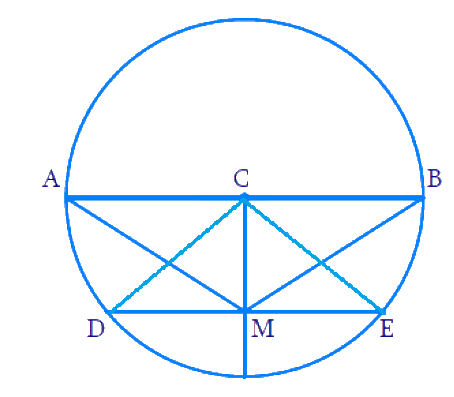# Ex.10.1 Q1 Circles Solution - NCERT Maths Class 9

Go back to  'Ex.10.1'

## Question

Fill in the blanks:

(i) The center of the circle lies in            of the circle. (exterior / interior)

(ii) A point, whose distance from the center of circle is greater than its radius lies in                 of the circle. (exterior / interior)

(iii) The longest chord of the circle is a                   of the circle

(iv) An arc is a                  when its ends are the ends of a diameter

(v) Segment of a circle is the region between an arc and                  of the circle

(vi) A circle divides the plane, on which it lies, in                  parts

Video Solution
Circles
Ex 10.1 | Question 1

## Text Solution

(i) The center of the circle lies in interior of the circle. (exterior / interior)

Reasoning:

The collection of all points in a plane, which are at a fixed distance from a fixed point in the plane, is called a circle. The fixed point is the center of the circle.

(ii) A point, whose distance from the center of circle is greater than its radius lies in exterior of the circle. (exterior / interior)

Reasoning:

The collection of all points in a plane, which are at a fixed distance from a fixed point in the plane is called a circle. The fixed point is the center of the circle. Fixed distance is the radius of the circle. Any point outside the circle will have a greater distance compared to the radius.

(iii) The longest chord of the circle is a diameter of the circle.Reasoning:

Let us check by drawing a random chord \begin{align} {DE}\end{align} and diameter \begin{align} {AB}\end{align} in the circle.

$$AC = CD = CE = BC =$$ radius

$$AB = 2 \times$$ radius

In \begin{align}\Delta\;{DCE},\; {DE}<{DC}+{CE}\end{align} (sum of two sides should be greater than the third side) .

\begin{align} &{DE}\;\lt\;r+r\\ &{DE}\,\lt\;2 r\\&{DE}\;\lt \;{AB}\\&{DE} \;\lt \;\text{diameter}\end{align}

Thus we know that any chord drawn randomly (without passing through the centre) will be shorter than the diameter. Thus Diameter is the longest chord in the circle.

(iv) An arc is a semicircle when its ends are the ends of a diameter.

Reasoning:

We know that diameter is the longest chord in the circle. And diameter divides the circle into $$2$$ equal halves or arcs. When two arcs are equal, each is a semicircle.

(v) Segment of a circle is the region between an arc and chord of the circle.

Reasoning:

The region between a chord and either of its arcs is called a segment of the circular region or simply a segment of the circle.

(vi) A circle divides the plane, on which it lies, in three parts.

Reasoning:

A circle divides the plane on which it lies into three parts.

They are:

(i) inside the circle, which is also called the interior of the circle;

(ii) the circle and

(iii) outside the circle, which is also called the exterior of the circle.

Video Solution
Circles
Ex 10.1 | Question 1

Learn from the best math teachers and top your exams

• Live one on one classroom and doubt clearing
• Practice worksheets in and after class for conceptual clarity
• Personalized curriculum to keep up with school